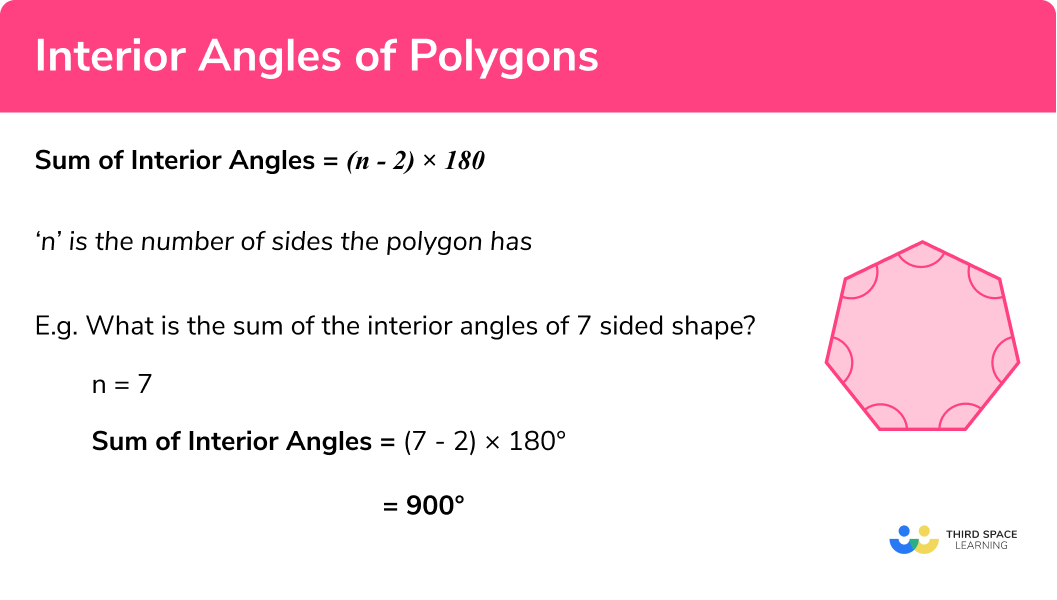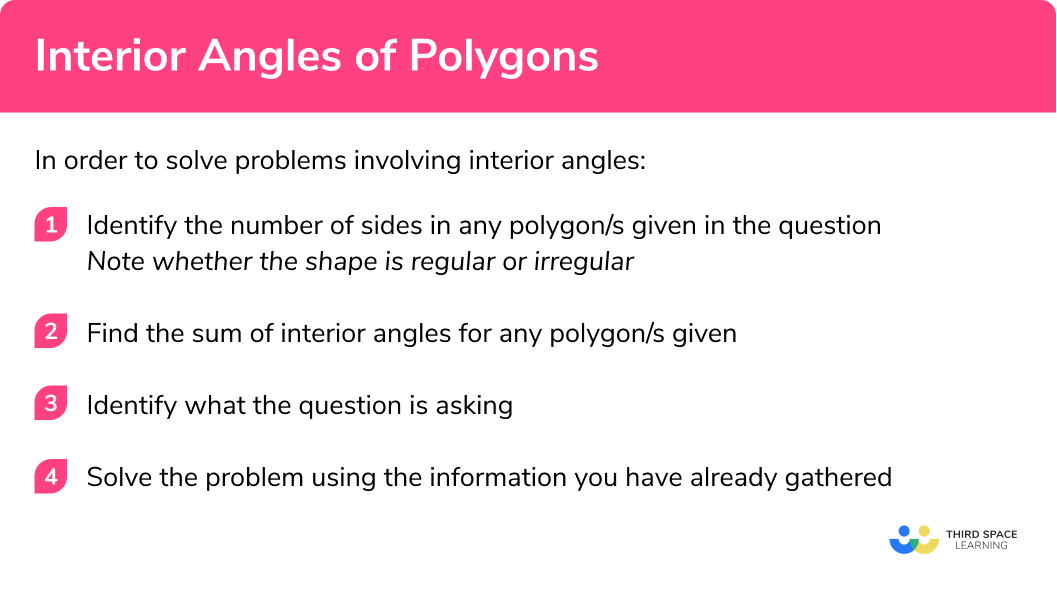# Interior Angles Of A Polygon

Here we will learn about interior angles in polygons including how to calculate the sum of interior angles for a polygon, single interior angles and use this knowledge to solve problems.

There are also angles in polygons worksheets based on Edexcel, AQA and OCR exam questions, along with further guidance on where to go next if you’re still stuck.

## What are interior angles?

Interior angles are the angles inside a shape. They are the angles within a polygon made by two sides:

Interior and exterior angles form a straight line – they add to 180°:

We can calculate the sum of the interior angles of a polygon by splitting it into triangles and multiplying the number of triangles by 180°.

E.g.

The number of triangles a polygon can be split into is always 2 less than the number of sides.

E.g.

A heptagon has 7 sides.

7 – 2 = 5, so we can split the heptagon into 5 triangles:

$5\times180^{\circ} = 900^{\circ}$

The general formula is:

Sum of Interior Angles = (n – 2) × 180°
n’ is the number of sides the polygon has

Step by step guide: Angles in polygons

### What are interior angles of polygons?### Keywords

• Polygon:
A polygon is a two dimensional shape with at least three sides, where the sides are all straight lines.
• Regular & irregular polygons:
A regular polygon is where all angles are equal size and all sides are equal length
E.g. a square
An irregular polygon is where all angles are not equal size and/or all sides are not equal length
E.g. a trapezium.

## How to solve problems involving interior angles

In order to solve problems involving interior angles:

1. Identify the number of sides in any polygon/s given in the question.
Note whether the shape is regular or irregular.
2. Find the sum of interior angles for any polygon/s given.
3. Identify what the question is asking.
4. Solve the problem using the information you have already gathered with use of the formulae interior angle \textbf{+} exterior angle \, \textbf{=} \; \bf{180^{\circ}} and Sum of exterior angles \, \textbf{=} \, \bf{360^{\circ}} if required

### How to solve problems involving interior angles of a polygon.### Related lessons on angles in polygons

Interior angles of a polygon is part of our series of lessons to support revision on angles in polygons. You may find it helpful to start with the main angles in polygons lesson for a summary of what to expect, or use the step by step guides below for further detail on individual topics. Other lessons in this series include:

## Interior angles examples

### Example 1: finding a single interior angle of a regular polygon

Find the size of each interior angle for a regular decagon.

1. Identify the number of sides in any polygon/s given in the question. Note whether this are regular or irregular shapes.

10 sides – regular shape.

2Find the sum of interior angles for any polygon/s given.

Sum of interior angles = (n – 2) × 180°

As a decagon has 10 sides:

n=10, so we can substitute n=10 into the formula.

Sum of interior angles of a decagon = (10 – 2) × 180°

Sum of  interior angles of a decagon = 8 × 180°

Sum of  interior angles of a decagon = 1440°

3Identify what the question is asking you to find.

The question is asking for ‘each interior angle’. This means the size of one interior angle.

4Solve the problem using the information you have already gathered with use of the formulae interior angle \textbf{+} exterior angle \, \textbf{=} \; \bf{180^{\circ}} and Sum of exterior angles \, \textbf{=} \, \bf{360^{\circ}} if required

We know the sum of the interior angles for this polygon is 1440°.

We know, as it is a regular polygon, that all the angles are of equal size.

Therefore we can find the size of each interior angle by dividing the sum of interior angles by the number of angles in the polygon:

$\text{Each interior angle} = \frac{1440}{10}$

The size of each interior angle is 144°.

Step-by-step guide: Substitution

### Example 2: finding a single interior angle of an irregular polygon

The diagram shows a polygon. Find the size of angle x.

Identify the number of sides in any polygon/s given in the question. Note whether these are regular or irregular shapes.

Find the sum of interior angles for any polygon/s given.

Identify what the question is asking you to find.

Solve the problem using the information you have already gathered with use of the formulae interior angle \textbf{+} exterior angle \, \textbf{=} \; \bf{180^{\circ}} and Sum of exterior angles \, \textbf{=} \, \bf{360^{\circ}} if required

### Example 3: finding the number of sides given the interior angle of a regular polygon

Each of the interior angles of a regular polygon is 140°. How many sides does the polygon have?

Identify the number of sides in any polygon/s given in the question. Note whether these are regular or irregular shapes.

Find the sum of interior angles for any polygon/s given.

Identify what the question is asking you to find.

Solve the problem using the information you have already gathered with use of the formulae interior angle \textbf{+} exterior angle \, \textbf{=} \; \bf{180^{\circ}} and Sum of exterior angles \, \textbf{=} \, \bf{360^{\circ}} if required

### Example 4: multiple shapes

Shown below are three congruent regular pentagons. Find angle y.

Identify the number of sides in any polygon/s given in the question. Note whether these are regular or irregular shapes.

Find the sum of interior angles for any polygon/s given.

Identify what the question is asking you to find.

Solve the problem using the information you have already gathered with use of the formulae interior angle \textbf{+} exterior angle \, \textbf{=} \; \bf{180^{\circ}} and Sum of exterior angles \, \textbf{=} \, \bf{360^{\circ}} if required

### Example 5: problem solving to find the number of sides

Shown below are sections of three identical regular polygons where AB, BC and CA are all sides of the polygons.

ABC is an equilateral triangle formed by placing the three larger polygons together.

Calculate the number of sides each regular polygon has.

Identify the number of sides in any polygon/s given in the question. Note whether these are regular or irregular shapes.

Find the sum of interior angles for any polygon/s given.

Identify what the question is asking you to find.

Solve the problem using the information you have already gathered with use of the formulae interior angle \textbf{+} exterior angle \, \textbf{=} \; \bf{180^{\circ}} and Sum of exterior angles \, \textbf{=} \, \bf{360^{\circ}} if required

### Example 6: problem solving to find the number of angles

Shown is a regular pentagon. Find y.

Identify the number of sides in any polygon/s given in the question. Note whether these are regular or irregular shapes.

Find the sum of interior angles for any polygon/s given.

Identify what the question is asking you to find.

Solve the problem using the information you have already gathered with use of the formulae interior angle \textbf{+} exterior angle \, \textbf{=} \; \bf{180^{\circ}} and Sum of exterior angles \, \textbf{=} \, \bf{360^{\circ}} if required

### Common misconceptions

• Miscounting the number of sides
• Misidentifying if a polygon is regular or irregular
• Dividing the sum of interior angles by the number of triangles created.
You should divide by the number of sides to find the size of one interior angle (for regular polygons only)
• Incorrectly assuming all the angles are the same size
• Misidentifying which angle the questions is asking you to calculate

### Practice interior angles of a polygon questions

1. Find the sum of interior angles for a polygon with 13 sides

2340^{\circ}1980^{\circ}4680^{\circ}3960^{\circ}Sum of Interior Angles = (n-2)\times180

In this case n=13 , so the calculation becomes 11 \times 180 .

2. Find the size of one interior angle for a regular quadrilateral

360^{\circ}180^{\circ}72^{\circ}90^{\circ}The sum of interior angles in a quadrilateral is 360^{\circ} . For a regular shape all the angles are the same size, so we divide 360 by 4 to arrive at the answer.

3. Find the size of one interior angle for a regular nonagon

360^{\circ}140^{\circ}180^{\circ}135^{\circ}The sum of interior angles in a nonagon is 1260^{\circ} . For a regular shape all the angles are the same size, so we divide 1260 by 9 to arrive at the answer.

4. Each of the interior angles of a regular polygon is 165^{\circ} . How many sides does the polygon have?

 20  sides22  sides24  sides26  sidesSum of Interior Angles = (n-2)\times180

With this in mind, we have 165n=(n-2) \times 180

Which simplifies to 15n = 360

So n=24

5. Each of the interior angles of a regular polygon is 160^{\circ} . How many sides does the polygon have?

 16  sides18  sides20  sides22  sidesSum of Interior Angles = (n-2)\times180

With this in mind, we have 160n=(n-2)\times180

Which simplifies to 20n = 360

So n=18

6. Four interior angles in a pentagon are each 115^{\circ} . Find the size of the other angle.

540^{\circ}80^{\circ}460^{\circ}305^{\circ}By using the formula,

Sum of Interior Angles = (n-2)\times180

We know that a pentagon has interior angles that add up to 540^{\circ} .

### Interior angles of a polygon GCSE questions

1. Work out the size of the angle labeled x .(3 marks)

(6-2) \times 180 = 720

(1)

80 + 55 + 280 + 25 + 162 = 602

(1)

720-602=118

(1)

2. The diagram below shows a regular decagon.(a) Work out the size of angle a .

(b) Work out the size of angle b.

(5 marks)

(10-2) \times 180 = 1440

(1)

1440 \div 10 = 144

(1)

144\times 2 = 288

(1)

360 – 288 = 72

(1)

72 \div 2=36

(1)

3. A regular polygon’s interior and exterior angles are in the ratio 9 : 1 . How many sides does the polygon have?

(4 marks)

180^{\circ} in ratio 9:1

180 \div 10=18, 18 \times 9=162, 18 \times 1= 18

(1)

One interior angle =162^{\circ}

(1)

\begin{aligned} 162n&=(n-2) \times 180 \\ 162n&=180n-360 \end{aligned}

18n=360

(1)

n=20

(1)

## Learning checklist

You have now learned how to:

• Use conventional terms for geometry e.g. interior angle
• Knowing names and properties of polygons
• Calculate the sum of interior angles for a regular polygon
• Derive and use the sum of angles in a triangle to deduce and use the angle sum in any polygon, and to derive properties of regular polygons
• Calculate the size of the interior angle of a regular polygon

## Still stuck?

Prepare your KS4 students for maths GCSEs success with Third Space Learning. Weekly online one to one GCSE maths revision lessons delivered by expert maths tutors.

Find out more about our GCSE maths tuition programme.